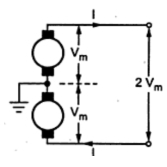### Comparison of Volume of Copper in Overhead System

The selection of a particular type of a.c. or d.c. system for the transmission and distribution is based on comparison of amount of material i.e. copper necessary for the various systems. As mentioned earlier, the maximum stress in the overhead system exists between the conductor and earth. Hence comparison of material required is done assuming the maximum voltage between any conductor and earth being the same. The assumptions made for the comparison are :
1. The power (P) transmitted by all the systems is same.
2. The distance ( l  ) over which the power is transmitted is same.
3. The power loss (W) in all the systems are same.
4. The maximum voltage (Vm) exists between any conductor and the earth, in all the systems.
Based on these assumptions, let us compare the various types of systems for the volume of copper required.
1.1 Two Wire D.C. System With One Line Earthed
The system is represented in the Fig. 1.Fig. 1

The maximum voltage between the conductors is Vm, as one material is earthed.

Where P = Power transmitted
Let R = resistance of each conductor

Total copper losses in both the lines are,

The volume of copper required for other systems is compared by taking volume of copper required for this system as base. Let it be constant K and volume of copper required for other systems can be expressed interms of K.

1.2 Two Wire D.C. System With Midpoint Earthed
The system is represented in the Fig. 2.Fig. 2

As power transmitted is same as P, the current in each conductor is,

The total copper loss in both the lines is,

where A = area of cross-section of each line.

The total volume of copper required is,

Thus the volume of copper required in this system is one fourth the volume of copper required for two wire d.c. system with one line earthed.
1.3 Three Wire D.C. System
The system is represented as shown in the Fig. 3.Fig. 3

When the load is balanced, current through the third neutral wire is zero.

Let A = Cross-section of outer conductors
Let area of cross-section of the middle neutral wire is half of the area of cross-section of the outer conductor.
Hence the total volume of copper
= Volume of copper for outer wires + volume of copper for neutral wire

Thus the volume of copper required in this system is 0.3125 times the volume of copper required for two wire d.c. system with one line earthed.
1.4 Three Phase Three Wire A.C. System
This is most commonly used system for the transmission. The three phase three wire star connected system with neutral earthed is shown in the Fig. 4.Fig. 4

The maximum voltage between each line conductor and the neutral is Vm as shown in the Fig. 4.
The R.M.S. value of the voltage per phase given by,

Then total power transmitted is P watts hence per phase power transmission is,

Let cosΦ be the load power factor

Hence the total copper loss is,

The volume of the copper required is

Thus the volume of copper required depends on power factor of the load and it is 0.5/cos2Φ times the volume of copper required by twp wire d.c. system with one line earthed. This system may be delta connected but irrespective of the method of connection star or delta, the result derived remains same.
1.5 Three Phase Four Wire A.C. System
This system is popularly used for secondary distribution. The neutral is also made available for the connection of the load. The system is shown in the Fig. 4.
Assuming the load balanced, there is no current flowing through the neutral.
The cross-section area of neutral is half the cross-section of each conductor i.e. 0.5 A where A is cross-section of each conductor.
The maximum voltage between any conductor and the neutral is Vm hence r.m.s. voltage per phase is,

The power transmitted per phase is,

Hence all the calculations upto the copper losses and expression of A remain same as derived for three  phase three wire system.

The total volume of copper requires is,
= Volume of copper for 3 lines + copper required for neutral

Thus the volume of copper required is 0.583/cos2Φ times the volume of copper required by two wire d.c. system with one line earthed.

#### 1 comment:

1.over head transmission lines planning, routing, and design and material supply within a stipulated time schedule is really must.